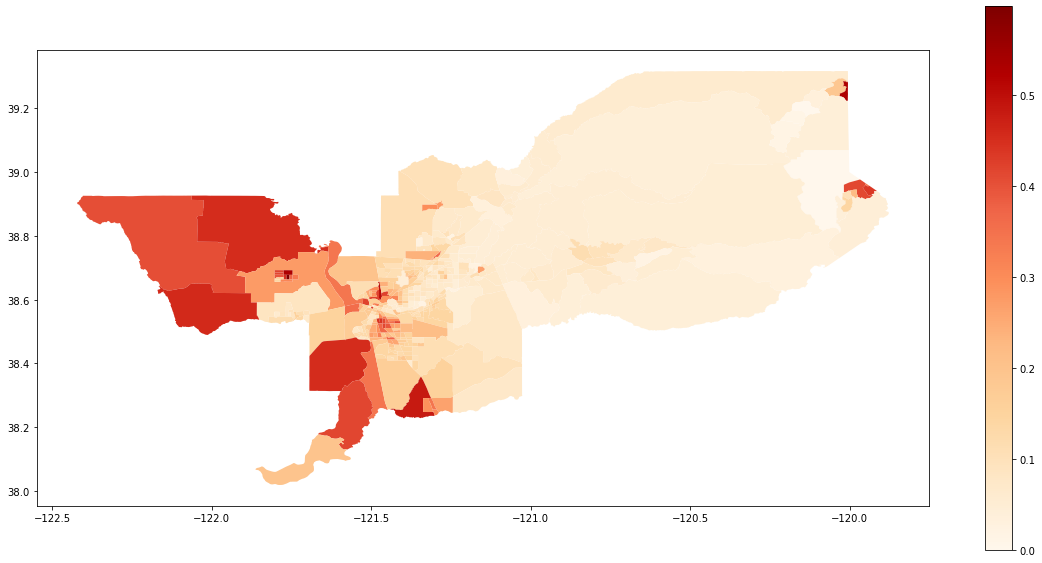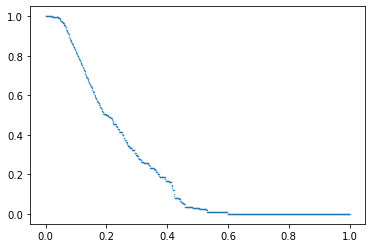aspatial_examples

# PySAL segregation module for aspatial indexes

This is an example notebook of functionalities for aspatial indexes of the segregation module. Firstly, we need to import the packages we need.

%matplotlib inline

import geopandas as gpd
import segregation
import libpysal


Then it's time to load some data to estimate segregation. We use the data of 2000 Census Tract Data for the metropolitan area of Sacramento, CA, USA.

We use a geopandas dataframe available in PySAL examples repository. We highlight that for nonspatial segregation measures only a pandas dataframe would also work to estimate.

s_map = gpd.read_file(libpysal.examples.get_path("sacramentot2.shp"))
s_map.columns

Index(['FIPS', 'MSA', 'TOT_POP', 'POP_16', 'POP_65', 'WHITE_', 'BLACK_',
'ASIAN_', 'HISP_', 'MULTI_RA', 'MALES', 'FEMALES', 'MALE1664',
'FEM1664', 'EMPL16', 'EMP_AWAY', 'EMP_HOME', 'EMP_29', 'EMP_30',
'EMP16_2', 'EMP_MALE', 'EMP_FEM', 'OCC_MAN', 'OCC_OFF1', 'OCC_INFO',
'HH_INC', 'POV_POP', 'POV_TOT', 'HSG_VAL', 'FIPSNO', 'POLYID',
'geometry'],
dtype='object')

The data have several demographic variables. We are going to assess the segregation of the Hispanic Population (variable 'HISP_'). For this, we only extract some columns of the geopandas dataframe.

gdf = s_map[['geometry', 'HISP_', 'TOT_POP']]


We also can plot the spatial distribution of the composition of the Hispanic population over the tracts of Sacramento:

gdf['composition'] = gdf['HISP_'] / gdf['TOT_POP']

gdf.plot(column = 'composition',
cmap = 'OrRd',
figsize=(20,10),
legend = True)

<matplotlib.axes._subplots.AxesSubplot at 0x2390c2ca550>## Notation

For consistency of notation, we assume that $n_{ij}$ is the population of unit $i \in \{1, ..., I\}$ of group $j \in \{x, y\}$, also $\sum_{j}n_{ij} = n_{i.}$, $\sum_{i}n_{ij} = n_{.j}$, $\sum_{i}\sum_{j}n_{ij} = n_{..}$, $\tilde{s}_{ij} = \frac{n_{ij}}{n_{i.}}$, $\hat{s}_{ij} = \frac{n_{ij}}{n_{.j}}$. The segregation indexes can be build for any group $j$ of the data.

### Dissimilarity

Introduced by Duncan, O. and B. Duncan (1955). A methodological analysis of segregation indexes. American Sociological Review 20, 210–17., the Dissimilarity Index (D) is given by:

$$D = \sum_{i=1}^{I}\frac{n_{i.}\mid \tilde{s}_{ij}-\frac{n_{.j}}{n_{..}}\mid}{2n_{..}\frac{n_{.j}}{n_{..}}\left ( 1-\frac{n_{.j}}{n_{..}} \right )}$$

and

$$0 \leqslant D \leqslant 1$$

The index is fitted below:

from segregation.aspatial import Dissim
index = Dissim(gdf, 'HISP_', 'TOT_POP')
type(index)

segregation.aspatial.aspatial_indexes.Dissim

All the segregation classes have the statistic and the core_data attributes. We can access the point estimation of D for the data set with the statistic attribute:

index.statistic

0.32184656076566864

The interpretation of this value is that 32.18% of the hispanic population would have to move to reach eveness in Sacramento.

### Gini

The Gini coefficient is given by:

$$G=\sum_{i_1=1}^{I}\sum_{i_2=1}^{I}\frac{n_{i_1.}n_{i_2.}\mid \tilde{s}_{ij}^{i_1}-\tilde{s}_{ij}^{i_2}\mid}{2n_{..}^2\frac{n_{.j}}{n_{..}}\left ( 1-\frac{n_{.j}}{n_{..}} \right )}$$

The index is fitted below:

from segregation.aspatial import GiniSeg
index = GiniSeg(gdf, 'HISP_', 'TOT_POP')
type(index)

segregation.aspatial.aspatial_indexes.GiniSeg
index.statistic

0.43506510676886234

### Entropy

The global entropy (E) is given by:

$$E = \frac{n_{.j}}{n_{..}} \ log\left ( \frac{1}{\frac{n_{.j}}{n_{..}}} \right )+\left ( 1-\frac{n_{.j}}{n_{..}} \right )log\left ( \frac{1}{1-\frac{n_{.j}}{n_{..}}} \right )$$

while the unit's entropy is analogously:

$$E_i = \tilde{s}_ {ij} \ log\left ( \frac{1}{\tilde{s}_ {ij}} \right )+\left ( 1-\tilde{s}_ {ij} \right )log\left ( \frac{1}{1-\tilde{s}_ {ij}} \right ).$$

Therefore, the entropy index (H) is given by:

$$H = \sum_{i=1}^{I}\frac{n_{i.}\left ( E-E_i \right )}{En_{..}}$$

The index is fitted below:

from segregation.aspatial import Entropy
index = Entropy(gdf, 'HISP_', 'TOT_POP')
type(index)

segregation.aspatial.aspatial_indexes.Entropy
index.statistic

0.09459760633014454

### Atkinson

The Atkinson index (A) is given by:

$$A = 1 - \frac{\frac{n_{.j}}{n_{..}}}{1-\frac{n_{.j}}{n_{..}}}\left | \sum_{i=1}^{I}\left [ \frac{\left ( 1-\tilde{s}_{ij} \right )^{1-b}\tilde{s}_{ij}^bt_i}{\frac{n_{.j}}{n_{..}}n_{..}} \right ] \right |^{\frac{1}{1-b}}$$

where $b$ is a shape parameter that determines how to weight the increments to segregation contributed by different portions of the Lorenz curve.

The index is fitted below (note you can modify the parameter b):

from segregation.aspatial import Atkinson
index = Atkinson(gdf, 'HISP_', 'TOT_POP', b = 0.5)
type(index)

segregation.aspatial.aspatial_indexes.Atkinson
index.statistic

0.15079259382667654

### Concentration Profile

The Concentration Profile (R) measure is discussed in Hong, Seong-Yun, and Yukio Sadahiro. "Measuring geographic segregation: a graph-based approach." Journal of Geographical Systems 16.2 (2014): 211-231. and tries to inspect the evenness aspect of segregation. The threshold proportion $t$ is given by:

$$\upsilon_t = \frac{\sum_{i=1}^{I}n_{ij}g(t,i)}{\sum_{i=1}^{I}n_{ij}}.$$

In the equation, $g(t, i)$ is a logical function that is defined as:

$$g(t,i) = \begin{cases} 1 & if \ \frac{n_{ij}}{n_{i.}} \geqslant t \\ 0 & \ otherwise. \end{cases}$$

The Concentration Profile (R) is given by:

$$R=\frac{\frac{n_{.j}}{n_{..}}-\left ( \int_{t=0}^{\frac{n_{.j}}{n_{..}}}\upsilon_tdt - \int_{t=\frac{n_{.j}}{n_{..}}}^{1}\upsilon_tdt \right )}{1-\frac{n_{.j}}{n_{..}}}.$$

The index is fitted below:

from segregation.aspatial import ConProf
index = ConProf(gdf, 'HISP_', 'TOT_POP')
type(index)

segregation.aspatial.aspatial_indexes.ConProf
index.statistic

0.1376874794741899

In addition, this index has a plotting method to see the profile estimated.

index.plot()

<matplotlib.collections.PathCollection at 0x2390c31c710>### Isolation

Isolation (xPx) assess how much a minority group is only exposed to the same group. In other words, how much they only interact the members of the group that they belong. Assuming $j = x$ as the minority group, the isolation of $x$ is giving by:

$$xPx=\sum_{i=1}^{I}\left ( \hat{s}_{ix} \right )\left ( \tilde{s}_{ix} \right ).$$

The index is fitted below:

from segregation.aspatial import Isolation
index = Isolation(gdf, 'HISP_', 'TOT_POP')
type(index)

segregation.aspatial.aspatial_indexes.Isolation
index.statistic

0.2319615486459151

The interpretation of this number is that if you randomly pick a hispanic person of a specific tract of Sacramento, there is 23.19% of probability that this member shares a unit with another hispanic.

### Exposure

The Exposure (xPy) of $x$ is giving by

$$xPy=\sum_{i=1}^{I}\left ( \hat{s}_{iy} \right )\left ( \tilde{s}_{iy} \right ).$$

The index is fitted below:

from segregation.aspatial import Exposure
index = Exposure(gdf, 'HISP_', 'TOT_POP')
type(index)

segregation.aspatial.aspatial_indexes.Exposure
index.statistic

0.7680384513540848

The interpretation of this number is that if you randomly pick a hispanic person of a specific tract of Sacramento, there is 76.8% of probability that this member shares a unit with an nonhispanic.

### Correlation Ratio

The correlation ratio (V or $Eta^2$) is given by

$$V = Eta^2 = \frac{xPx - \frac{n_{.x}}{n_{..}}}{1 - \frac{n_{.x}}{n_{..}}}.$$

The index is fitted below:

from segregation.aspatial import CorrelationR
index = CorrelationR(gdf, 'HISP_', 'TOT_POP')
type(index)

segregation.aspatial.aspatial_indexes.CorrelationR
index.statistic

0.09164042012926693

### Modified Dissimilarity

The Modified Dissimilarity Index (Dct) based on Carrington, W. J., Troske, K. R., 1997. On measuring segregation in samples with small units. Journal of Business & Economic Statistics 15 (4), 402–409, evaluates the deviation from simulated evenness. This measure is estimated by taking the mean of the classical $D$ under several simulations under evenness from the global minority proportion.

Let $D^*$ be the average of the classical D under simulations draw assuming evenness from the global minority proportion. The value of Dct can be evaluated with the following equation:

$$Dct = \begin{cases} \frac{D-D^*}{1-D^*} & if \ D \geqslant D^* \\ \frac{D-D^*}{D^*} & if \ D < D^* \end{cases}$$

The index is fitted below (note you can change the number of simulations):

from segregation.aspatial import ModifiedDissim
index = ModifiedDissim(gdf, 'HISP_', 'TOT_POP', iterations = 500)
type(index)

segregation.aspatial.aspatial_indexes.ModifiedDissim
index.statistic

0.31075096050008966

### Modified Gini

The Modified Gini (Gct) based also on Carrington, W. J., Troske, K. R., 1997. On measuring segregation in samples with small units. Journal of Business & Economic Statistics 15 (4), 402–409, evaluates the deviation from simulated evenness. This measure is estimated by taking the mean of the classical G under several simulations under evenness from the global minority proportion.

Let $G^*$ be the average of G under simulations draw assuming evenness from the global minority proportion. The value of Gct can be evaluated with the following equation:

$$Gct = \begin{cases} \frac{G-G^*}{1-G^*} & if \ G \geqslant G^* \\ \frac{G-G^*}{G^*} & if \ G < G^* \end{cases}$$

The index is fitted below (note you can change the number of simulations):

from segregation.aspatial import ModifiedGiniSeg
index = ModifiedGiniSeg(gdf, 'HISP_', 'TOT_POP', iterations = 500)
type(index)

segregation.aspatial.aspatial_indexes.ModifiedGiniSeg
index.statistic

0.4217880747799781

### Bias-Corrected Dissimilarity

The Bias-Corrected Dissimilarity (Dbc) index is presented in Allen, R., Burgess, S., Davidson, R., Windmeijer, F., 2015. More reliable inference for the dissimilarity index of segregation. The econometrics journal 18 (1), 40–66. The Dbc is given by:

$$D_{bc} = 2D - \bar{D}_{b}$$

where $\bar{D}_b$ is the average of $B$ resampling using the observed conditional probabilities for a multinomial distribution for each group independently.

The index is fitted below (note you can change the value of B):

from segregation.aspatial import BiasCorrectedDissim
index = BiasCorrectedDissim(gdf, 'HISP_', 'TOT_POP', B = 500)
type(index)

segregation.aspatial.aspatial_indexes.BiasCorrectedDissim
index.statistic

0.32137576283345015

### Density-Corrected Dissimilarity

The Density-Corrected Dissimilarity (Ddc) index is presented in Allen, R., Burgess, S., Davidson, R., Windmeijer, F., 2015. More reliable inference for the dissimilarity index of segregation. The econometrics journal 18 (1), 40–66. The Ddc measure is given by:

$$D_{dc} = \frac{1}{2}\sum_{i=1}^{I} \hat{\sigma}_{i} n\left ( \hat{\theta}_i \right )$$

where

$$\hat{\sigma}^2_i = \frac{\hat{s}_{ix} (1-\hat{s}_{ix})}{n_{.x}} + \frac{\hat{s}_{iy} (1-\hat{s}_{iy})}{n_{.y}}$$

and $n\left ( \hat{\theta}_i \right )$ is the $\theta_i$ that maximizes the folded normal distribution $\phi(\hat{\theta}_i-\theta_i) + \phi(\hat{\theta}_i+\theta_i)$ where

$$\hat{\theta_i} = \frac{\left | \hat{s}_{ix}-\hat{s}_{iy} \right |}{\hat{\sigma_i}}.$$

and $\phi$ is the standard normal density.

The index is fitted below (note you can change the tolerance of the optimization step):

from segregation.aspatial import DensityCorrectedDissim
index = DensityCorrectedDissim(gdf, 'HISP_', 'TOT_POP', xtol = 1e-5)
type(index)

segregation.aspatial.aspatial_indexes.DensityCorrectedDissim
index.statistic

0.295205155464069

### Minimum-Maximum Index (MM)

The Minimum-Maximum Index (MM) is an abstraction of the aspatial version of O'Sullivan, David, and David WS Wong. "A surface‐based approach to measuring spatial segregation." Geographical Analysis 39.2 (2007): 147-168. Its formula is given by:

$$MM = \frac{\sum_{i=1}^{n} max \left ( \hat{s}_{i1}, \hat{s}_{i2} \right ) - \sum_{i=1}^{n} min \left ( \hat{s}_{i1}, \hat{s}_{i2} \right )}{\sum_{i=1}^{n} max \left ( \hat{s}_{i1}, \hat{s}_{i2} \right )}$$

where the sub-indexes $1$ and $2$ are two mutually exclusive groups (for example, Hispanic and non-Hispanic population). Internally, segregation creates the complementary group of HISP_.

from segregation.aspatial import MinMax
index = MinMax(gdf, 'HISP_', 'TOT_POP')
type(index)

segregation.aspatial.aspatial_indexes.MinMax
index.statistic

0.4869650840249441